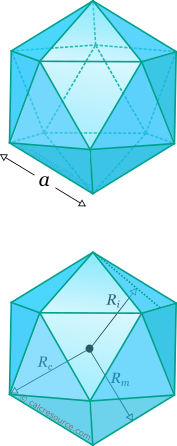## Properties of Icosahedron

This tool calculates the basic geometric properties of a regular icosahedron. Enter the shape dimension 'a' or 'h' below. The calculated results will have the same units as your input. Please use consistent units for any input.

 Known data: Edge length Inradius Circumradius Midradius Geometric properties: Volume = Surface area = Face area = Edge length = Inradius = Circumradius = Midradius = Dihedral angle (°) = Number of faces = Number of edges = Number of vertices =## Definitions

### Geometry

Icosahedron is a regular polyhedron with twenty faces. By regular is meant that all faces are identical regular polygons (equilateral triangles for the icosahedron). It is one of the five platonic solids (the other ones are tetrahedron, cube, octahedron and dodecahedron). It has 20 faces, 30 edges and 12 vertices.

The volume of a regular icosahedron is given by the formula:

where the edge length.

The total surface area, is given by the following formula:

where is the surface area of one face of the icosahedron.

The dihedral angle is defined as the interior angle between two adjacent faces of the polyhedron. For the regular icosahedron it is given by the expression:

where is the golden ratio.

For any regular polyhedron, three spheres can be commonly defined: one that passes through all the vertices, called circumscribed sphere or circumsphere, one that passes through the centroids of all faces, called inscribed sphere or insphere, and one that passes through the middles of all edges, called midsphere. The radii of these spheres, circumradius , inradius and midradius , respectively, can be found for a regular icosahedron, through the following expressions: